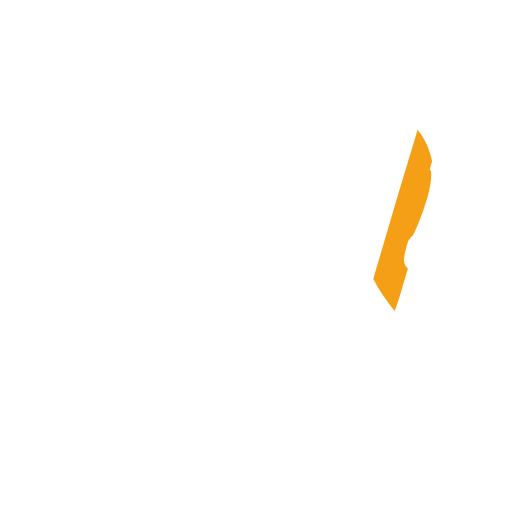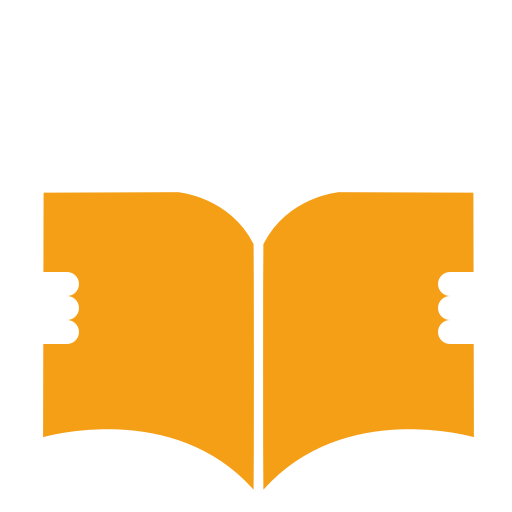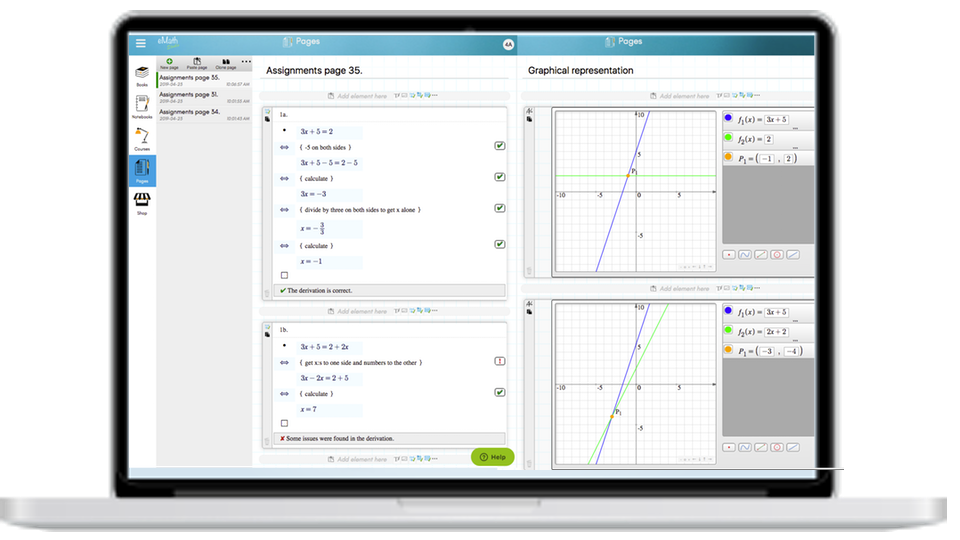# eMath StudioThe only math notebook where you can check the correctness of your own calculations automaticallyMath Tools
Great tools with automatic step-by-step checkingOther Tools
Embed web pages, videos, sound files and add images.# Write your assignments as loose notes or in notebooks

eMath Studio Notebooks are perfect for taking individual notes. Students can take notes during class, teachers can use it for creating course material, and project groups can use it as a central storage of content. You can create an unlimited number of personal digital notebooks in eMath Studio. A notebook is organised into chapters, sections, and pages. The list of content in a notebook is automatically updated. The overall structure of the notebook can be edited directly by manipulating the list of contents.

### Math Tools

MATH EDITOR WITH IN-LINE CALCULATOR

## WRITE EASILY

The most popular editor is the structured derivations editor, where students can build word problems easily or perform longer calculations. There are places for inserting assumptions and defining variables. In addition, between each calculation step there is space to explain in your own words what you are doing. These verbal explanations are extremely important when trying to remember or understand what happened in the calculation step. Mathematical symbols are entered by selecting them from a palette, or by writing simple shorthand keywords.GRAPHS AND TABLES

## VISUALIZE AND DRAW

With the different visualization tools, you can draw geometric figures, plot function graphs, or create tables with mathematical content as well as diagrams for statistical presentations.

AUTOMATIC CHECKING

## CHECK. CORRECT. SUBMIT.

When you are finished with your calculations, or when you are uncertain of a step, you can check to see if you have calculated correctly. For each step, you will get a correct mark or an exclamation mark telling you where you might have made a mistake.### Other Useful Tools

TEXT, AUDIO, IMAGES AND EMBEDDING